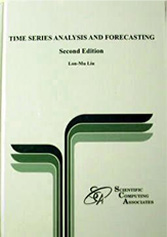TEL:
+708-771-4567
FAX:
+708-771-4569
EMAIL:(April 09, Version 2.2)
By Lon-Mu Liu, Ph.D., Univ. of Illinois at Chicago

ISBN: 978-0-9765056-8-6  Format: Hardcover; 578 pp

This book is one of the most important works written on applied time series analysis and forecasting. The author achieves this goal by distilling and integrating important research results on time series analysis and forecasting into cohesive and comprehensible methodologies.
Types of a time series Applications of time series analysis Approaches for time series analysis and its applications Model building and forecasting Principle of parsimony Automatic model identification approaches Evaluation of forecast performance Effects of outliers on forecast performance

References Stationary time series and their characterization Autocorrelation function Partial autocorrelation function Extended autocorrelation function Stationary time series models and their characteristics Autoregressive, moving average, and mixed ARMA models Nonstationary time series models and their characteristics Model building Model identification Model estimation Diagnostic checking Forecasting Illustrative examples

Attributes of seasonal time series Stationary seasonal models and their characteristics Pure seasonal autoregressive, moving average, and mixed ARMA models Nonstationary seasonal models and their characteristics Multiplicative and nonmultiplicative seasonal models Seasonal model identification Estimation of seasonal models Diagnostic checking of seasonal models Forecasting with seasonal models Illustrative examples

Two forms of ARIMA models Automatic identification of ARIMA models for nonseasonal time series Automatic identification of ARIMA models for seasonal time series Identification of seasonal ARIMA models using a filtering method Illustrative examples

Relationship of transfer function models to regression models Multiple-input transfer function models Transfer function model identification using the LTF method Transfer function model estimation Diagnostic checking transfer function models Forecasting with transfer function models Illustrative examples

Trading day effects Holiday effects Modeling trading day effects using ARIMA models Modeling holiday effects using ARIMA models Identification of an ARIMA model for a time series with calendar effects Illustrative examples

Characterizations for intervention effects Modeling strategies for intervention analysis Forecasting with an intervention model Outliers in time series and their types (AO, IO, LS, TC) Methods for outlier detection and adjustment An iterative procedure for joint estimation of model parameters and outlier effects Intervention analysis in the presence of outliers Forecasting in the presence of outliers Handling outliers at the end of a time series Handling missing data in a time series Illustrative examples

Naive and averaging methods Simple (single) exponential smoothing Double exponential smoothing Holt's two parameter exponential smoothing Winters' additive and multiplicative smoothing methods General exponential smoothing using seasonal indicators General exponential smoothing using harmonic functions Relating exponential smoothing to Box-Jenkins ARIMA models

Concepts in data mining Application of data mining concepts on time series analysis and forecasting The role of expert system time series modeling in data mining Time series data mining on electricity loads An example of time series data mining in business operations Methodology for data mining and knowledge discovery for time series Using automatic outlier detection methods as a tool for time series data mining

Types of transformations for time series Power transformation and retransformation Effects of transformation on forecasts Debiasing forecasts in retransformation Procedures for searching the optimal power transformation in forecasting applications Remarks on power transformation Illustrative examples

Symmetric ARCH, GARCH, IGARCH, and GARCH-M models Asymmetric GJR-GARCH, EGARCH, and Threshold GARCH models Non-Normal error distributions based on Student-t, Cauchy, and GED Measuring volatility leverage effects and risk premium Illustrative examples

Periodic grouping of data based on calendar and threshold values Model estimation using the weighted method Time-segmented ARIMA models for seasonal time series Value-segmented threshold autoregressive (TAR) models Multiplicative TAR models Multiplicative threshold ARIMA models Time-segmented transfer function models Threshold transfer function models Handling clustered large outliers by discounting segments of time series data Illustrative examples

Threshold autoregressive (TAR) models Nonlinearity TAR-F Test Identification of threshold values Illustrative examples

Vector ARMA models Relationship between vector ARMA models and other time series models Characteristics of some vector ARMA models Partial autoregression matrices in vector models Extended cross correlation matrices (ECCM) Building vector ARMA models Forecasting using vector ARMA models Multiplicative seasonal vector ARMA models Eigenvalue-eigenvector analyses in multivariate time series Alternative approaches to modeling multivariate time series Illustrative examples

Simultaneous transfer function models Structural form and reduced form Models Model building strategy for reduced form STF models Model building strategy for structural form STF models Multivariate time series analysis and forecasting with interventions Econometric modeling using STF models Illustrative examples

Dynamic relationships between economic time series Granger causality Causality testing using vector ARMA models A decision tree approach for detecting dynamic relationships Other procedures for testing causality (Sims test and Haugh test) Illustrative examples